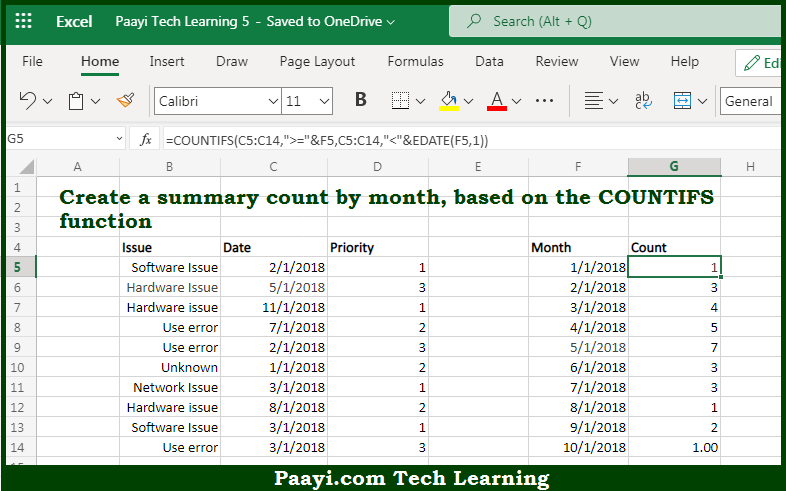# Learn How to Create Summary COUNT by Month With COUNTIFS in Microsoft Excel

Written by | 0 Comments | 632 Views

In this article, you will learn how to COUNT various things in Microsoft Excel using a single or combination of functions and its purpose. You will also get to know how to Create Summary COUNT by Month With COUNTIFS and see the generic formula.

Create Summary COUNT by Month With COUNTIFS in Microsoft Excel

The main purpose of this formula is to create a summary count by month. Here we will learn how to create a summary count by month with the COUNTIFS function in Microsoft Excel. That implies, with the help of a formula based on the COUNTIF and EDATE function you can able to create a summary count by month. So, with the help of this formula, you can able to create a summary count by a month with the COUNTIFS function.

General Formula to Create Summary COUNT by Month With COUNTIFS

=COUNTIFS(dates,">="&A1,dates,"<"&EDATE(A1,1))

The Explanation for the Create Summary COUNT by Month With COUNTIFSSo we know that with the help of the given formula above you can able to create a summary count by month. Here we will learn how to create a summary count by month with the COUNTIFS function in Microsoft Excel. As we know that here we have to use the COUNTIFS function to generate a count. The first column of the summary table as given here is a date for the first of each month in the year. We need to supply criteria, to generate a total count per month that will clear all the issues that appear every month. Since we have actual dates in the given column, we can construct the criteria we need using the date itself, and a second date created with the EDATE function. So, with the help of this formula, you can able to create a summary count by month.